Courses

# Test: The Ideal Diode

## 10 Questions MCQ Test Electronic Devices | Test: The Ideal Diode

Description
This mock test of Test: The Ideal Diode for Electrical Engineering (EE) helps you for every Electrical Engineering (EE) entrance exam. This contains 10 Multiple Choice Questions for Electrical Engineering (EE) Test: The Ideal Diode (mcq) to study with solutions a complete question bank. The solved questions answers in this Test: The Ideal Diode quiz give you a good mix of easy questions and tough questions. Electrical Engineering (EE) students definitely take this Test: The Ideal Diode exercise for a better result in the exam. You can find other Test: The Ideal Diode extra questions, long questions & short questions for Electrical Engineering (EE) on EduRev as well by searching above.
QUESTION: 1

### For an ideal diode which of the following is true?

Solution:

Both of the facts mentioned hold true for an ideal operational amplifier.

QUESTION: 2

Solution:

Vd = Vi – Vo.

QUESTION: 3

### The figure below shows a circuit for charging a 12-V battery. If Vs is a sinusoid with 24-V peak amplitude, the fraction of each cycle during which the diode conducts is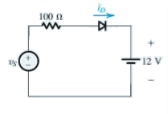Solution:

For one half of the cycle the diode conducts when 24 cos(Θ) = 12 or Θ is 600. Hence for a complete cycle the diode does not conducts for 2Θ or 1200. Hence the diode does not conducts for a third of a cycle.

QUESTION: 4

Diodes can be used in the making of

Solution:

Diodes are used to make rectifiers (full wave or half wave rectifiers are the most common examples), LED lamps uses diodes (diodes are generally doped for their use in this purpose) and logic gates can also be made using diodes using the fact that diodes are conducting only in the forward biased configuration.

QUESTION: 5

For the connections shown below, the equivalent logic gate is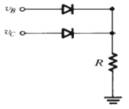Solution:

The following circuit behaves as a OR logic gate.

QUESTION: 6

For the connections shown below, the equivalent logic gate is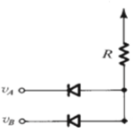Solution:

The circuit shown behaves as an AND logic gate.

QUESTION: 7

The figure below shows a circuit for an AC voltmeter. It utilizes a moving-coil meter that gives a full-scale reading when the average current flowing through it is 1 mA. The moving-coil meter has a 50-Ω resistance. The value of R that results in the meter indicating a full-scale reading when the input sine-wave voltage VI is 20 V peak-to-peak is​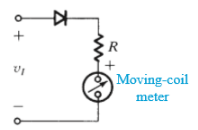Solution:

Average value of input voltage= 20/π or 6.366 V.
Since there is diode half of the time there is no voltage across the voltmeter hence the average value of the input voltage is 10/π or 3.183V.
The total resistance required to generate 1mA current is 3.183 kΩ out of which 50Ω is provided by the moving coil. Therefore, a resistance of 3.133 kΩ is required.

QUESTION: 8

The value of I and V for the circuit shown below are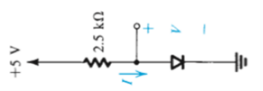Solution:

I = V/R or 5/2.5 or 2A
For an ideal diode when it is conducting the potential drop across its terminal is zero.

QUESTION: 9

The units frequently used to measure the forward bias and reverse bias current of a diode are

Solution:

The currents in forward bias is generally in MA and in the reverse bias it is very small and is generally measured in µA.

QUESTION: 10

A diode

Solution:

Both the statements are true for a diode.